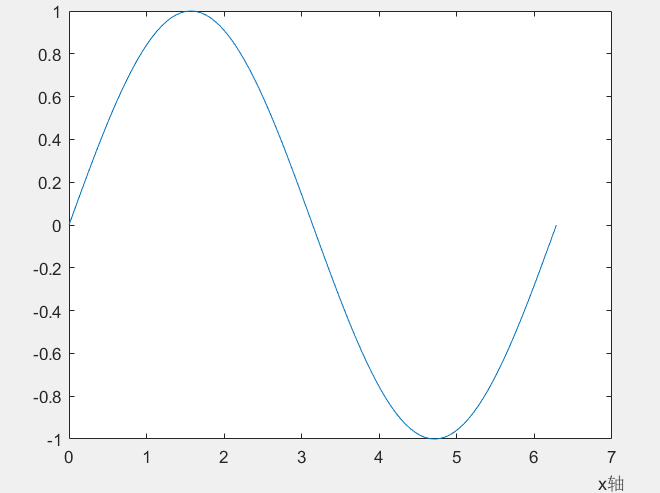• ## matlabxlabel位置设置

千次阅读 2020-03-20 17:54:40
xlabel(‘time’,‘FontSize’,12); 如果没有设置位置，默认是在中间 在xlabel中也有position用法 xlabel(‘time’,‘position’,[900,1870],‘FontSize’,12); 此时‘time’在你设置的位置 还有一种用法是类似图像...
xlabel(‘time’,‘FontSize’,12);
如果没有设置位置，默认是在中间
在xlabel中也有position用法
xlabel(‘time’,‘position’,[900,1870],‘FontSize’,12);
此时‘time’在你设置的位置
还有一种用法是类似图像的用法
pos=axis;%取得当前坐标轴的范围，即[xmin xmax ymin ymax]
xlabel(‘time’,‘FontSize’,12, ‘Position’,[pos(2) pos(3)])
x=0:pi/50:2*pi;
y=sin(x);
plot(x,y);
pos=axis;%取得当前坐标轴的范围，即[xmin xmax ymin ymax]
xlabel('x轴','position',[pos(2) 1.15*pos(3)]);%设置x轴标签的文本在图的右下方，1.15这个值根据自己的需要可以调整
形成的图参考链接2：http://blog.sina.com.cn/s/blog_7e6270010101nt0r.html#commonComment


展开全文• Matlab希腊字母Latex输入_...数据图 gnuplot: matlab: origin: 关于 TeX 和 LaTeX 的插图方面可以关参考...0.000000 font "" set xlabel "X axis" -5.000000,-2.000000 font "......\\Large f(x)=\\int_{-\\infty}^x...
Matlab希腊字母Latex输入_数学_自然科学_专业资料。1 I. TEX ...数据图 gnuplot: matlab: origin: 关于 TeX 和 LaTeX 的插图方面可以关参考...0.000000 font "" set xlabel "X axis" -5.000000,-2.000000 font "......\\Large f(x)=\\int_{-\\infty}^x e^{-t^2} 复制代码 方法二:使用 Matlab 的 latex()函数 比如要显示 sin(x)*exp(x^2+y^2) 则先在 Matlab 中输入......在 Matlab 支持的 LaTex 字符串中,用\\bf , \\it , \\rm 控制字符分别定义黑体、 斜体和正体字符, LaTex 字符串控制部分要加大括号 受 {}括起来。例如,text(......在 Matlab 支持的 LaTex 字符串中,用\\bf , \\it , \\rm 控制字符分别定义黑体、 斜体和正体字符, 受 LaTex 字符串控制部分要加大括号 {}括起来。例如,text(......数学软件 MATLAB绘图二 9.1 三维图形的精细处理 9.2...中除使用标准的ASCII字符 外,还可使用LATEX格式的...xlabel('x-axis'),ylabel('y-axis'),zlabel('......其中, 说明文字中除使用标准的ASCII字符外,还可使 用LATEX格式的控制字符。 除...'Label','&Quit','Call','close(gcf)'); 8.1.3 快捷菜单在MATLAB中,......其中说明文字中除使用标准的ASCII字符外,还可使用 LaTeX格式的控制字符。 除公共...例8-7 利用曲面对象绘制三维曲面z=sin(x)。 Matlab仿真技术与应用 8.4 对象......在 Matlab 支持的 LaTex 字符串中,用\\bf , \\it , \\rm 控制字符分别定义黑体、 斜体和正体字符, 受 LaTex 字符串控制部分要加大括号 {}括起来。例如,text(......还可使用 LaTeX 格式的控 制字符,这样就可以在图形上添加希腊字母、数学符号及...meshgrid命令为了绘制三维立体图形,MATLAB的方法是将x方向划分为m份,将y方向划分......图形修饰与控制 图形标注(title xlabel/ylabel legend text/gtext) LaTeX 格式字符控制 坐标、网格与边界控制(axis([xmin xmax ymin ymax]) axis auto axis ......由于 Latex 属于免费开源的软件,因此 MATLAB 兼容了 latex 格式, 以下所有的操作都是在 matlab 中进行的,是 matlab 环境下使用了 latex 语句而已 不来虚的了,......? ? ? extension: EPS Matlab OriginPro MS Visio GNUPlot SigmaPlot ? Latex pgfplots & Ticks Mfpic, TeXdraw, curve2e, feyn, feynMF, axodraw … page......('interpreter','latex','string', ['$$\\theta_{\\rm{bar}}=\\;$$' num2str(b)], 'position',[4.5,-4.0]); hold off xlabel('x [kpc]'); ......(续) 第十步:添加文本注释 >> xlabel('X Axis');ylabel('Y Axis');zlabel('Function Value'); >> title('Peaks') 4.7 保存和输出图形 MATLAB 提供了将......(x)=\\lim_{\\Delta x\\to 0}\\frac{k(x)-k(x-\\Delta x)}{\\Delta x}(后文再详细介绍在 matlab 中 的显示方式,包括 showLatex 这个函数。 )代码前后要......[pi/2-.1,-1.1]); latextext('$\\pi$',[pi-.1,-1.1]); set(gca,'xtick',[-pi -pi/2 0 pi/2 pi]); set(gca,'xticklabel',{'' '' ......(x,y2); hold off 4.1.3 设置曲线样式 MATLAB...xlabel(x轴说明) ylabel(y轴说明) text(x,y,图形...还可使用LaTeX格式的控制字符,这样就可以在图 形上......所有标注中均可使用汉字 % 对于特殊符号,如希腊字母,箭头等需要采用LaTeX格式 ...三维曲面的绘制 Matlab绘制三维曲面的命令有: (1)mesh(x,y,z)——绘制三维......第五章 MATLAB绘图 14.11.2020 精品课件 二维数据...xlabel(x轴说明) ylabel(y轴说明) text(x,y,图形...还可使用LaTeX格式的控制 字符,这样就可以在图形上......
展开全文• 标注x，y和z轴语法xlabel('string')xlabel(fname)xlabel(...,'PropertyName',PropertyValue,...)xlabel(axes_handle,...)h = xlabel(...)ylabel(...)ylabel(axes_handle,...)h = ylabel(...)zlabel(...)zlabel(axes_...
标注x，y和z轴语法xlabel('string')xlabel(fname)xlabel(...,'PropertyName',PropertyValue,...)xlabel(axes_handle,...)h = xlabel(...)ylabel(...)ylabel(axes_handle,...)h = ylabel(...)zlabel(...)zlabel(axes_handle,...)h = zlabel(...)描述对x、y和z轴图形对象都可以有一个标签。对二维坐标系而言，标签出现在各自代表轴的下方，对三维坐标系而言，标签出现在各自代表轴的旁边或者下方。xlabel('string')：表示在当前坐标轴的x轴进行标注xlabel(fname)：计算函数fname，此函数必然返回一个字符串。然后将此字符串标注在x轴旁边。xlabel(...,'PropertyName',PropertyValue,...)：为x轴所创建的文本图形对象指定属性名和属性值对。xlabel(axes_handle,...), ylabel(axes_handle,...), and zlabel(axes_handle,...)：在带axes_handle句柄的轴绘制而不是在当前轴h = xlabel(...), h = ylabel(...), and h = zlabel(...)：返回用作标签的文本对象的句柄。ylabel(...) and zlabel(...)：在当前轴分别标注y、z轴。备注增加一个xlabel, ylabel或者zlabel命令会替换掉旧标签。对三维图形而言，标签被放在前边或者旁边以便不会被隐藏。示例使用多元单行阵列为x轴创建多个标签：xlabel({'first line';'second line'})
展开全文• 展开全部Matlab中巧用LaTex众所周知，大多数科研工作者(大Boss或者象e68a8462616964757a686964616f31333337376264我们一样的学术打工仔)都会用到Matlab生成图片，图片的title或者legend最好有相应的说明，经常用到...
展开全部Matlab中巧用LaTex众所周知，大多数科研工作者(大Boss或者象e68a8462616964757a686964616f31333337376264我们一样的学术打工仔)都会用到Matlab生成图片，图片的title或者legend最好有相应的说明，经常用到公式，比方说α，β等。但大多数人往往是利用图像后处理软件如Photoshop或illustrator等对生成的图片进行加工。Latex是被公认的生成公式最漂亮的排版语言(软件)，成为事实上学术排版的标准。那么能否利用latex生成公式插入到Matlab生成的图片中呢？答案是肯定的。Matlab带有Latex解析器，能支持latex的各种符号，比如积分符号等等。Matlab图形中title、xlabel、ylabel、zlabel、textbox和legend等的Interpreter属性有三个属性：latex 、tex、none。默认为tex。当键入：>> set(text,'Interpreter')Matlab将返回'Interpreter'所包含的属性值：[ latex | {tex} | none ]。利用Matlab文本的Interpreter属性使我们能在图形中显示一个较为复杂的公式，例如在公式中除了有希腊字母外，还有分号、根号等数学符号。Tex的用法在Matlab的帮助文档里有详细介绍，这里主要介绍一下如何采用latex编辑公式。在matlab中，Latex编辑公式的基本格式：1、$$LaTeX命令$$2、$LaTeX命令$3、$$LaTeX命令$$1. 在图象中直接加字符，很简单。text('Interpreter','latex','String','$$\sqrt{x^2+y^2}$$','Position',[.5.5],… 'FontSize',16);2.  在legend里加数学字符h=legend('$$\sqrt{x^2+y^2}$$');set(h,'Interpreter','latex')当然也可以使用命令。以此类推也可以对title、xlabel、ylabel、zlabel和legend等使用LaTeX命令，如：xlabel({'$\int_0^x\!\int_y dF(u,v)$'},'Interpreter','latex')至于LaTeX命令使用方法可以参考LaTeX教程。另外，Matlab可以吧计算结果转化成Latex格式，对于Matlab计算出的符号运算结果，可以通过latex()函数转化成LeTeX命令格式。由于latex()函数只对符号表达式进行转换，对于数值结果一定要通过sym()函数转化成符号结果。所以，为防止对数值结果转化出错，可同时使用latex()和sym()函数：latex(sym(s)); 其中s代表符号表达式。例如：>>syms a b cs=a/b+c使用latex(s)后转化为LeTeX命令：{\frac {a}{b}}+cTex字符在输出一些数学公式时经常使用，它只能由类型为text的对象创建。函数title、xlabel、ylabel、zlabel或text都能 创建一个text对象，因此Tex字符转义符(带“\”的字符串)经常作为这些函数的输入参数。如果要输出希腊字母，可以使用texlabel函数将希腊 字母的变量名转化为希腊字母的函数，供函数title、xlabel、ylabel、zlabel或text使用。texlabel转换MATLAB表达式为等价的Tex格式字符串。它处理希腊字母的变量名为实际显示的希腊字母字符串。希腊字母的变量名为“\”后面的字符串。Tex字符及其函数表函数字符        代表符号        函数字符        代表符号        函数字符        代表符号\alpha        α        \upsilon        υ        \sim        ~\beta        β        \phi        ϕ        \leq        ≤\gamma        γ        \chi        χ        \infty        ∞\delta        δ        \psi        ψ        \clubsuit\epsilon        ϵ        \omega        ω        \diamondsuit\zeta        ζ        \Gamma        Γ        \heartsuit\eta        η        \Delta        Δ        \spadesuit\theta        θ        \Theta        Θ        \leftrightarrow        ↔\vartheta        ϑ        \Lambda        Λ        \leftarrow        ←\iota        ι        \Xi        Ξ        \uparrow        ↑\kappa        κ        \Pi        Π        \rightarrow        →\lambda        λ        \Sigma        Σ        \downarrow        ↓\mu        μ        \Upsilon        Υ        \circ        °\nu        ν        \Phi        Φ        \pm        ±\xi        ξ        \Psi        Ψ        \geq        ≥\pi        π        \Omega        Ω        \propto        ∝\rho        ρ        \forall        ∀        \partial        ∂\sigma        σ        \exists        ∃        \bullet        ∙\varsigma        ς        \ni                 \div        ÷\tau        τ        \cong        ≅        \neq        ≠\equiv        ≡\approx        ≈        \aleph        ℵ\Im                 \Re                 \wp\otimes        ⊗        \oplus        ⊕        \oslash\cap        ∩        \cup        ∪        \supseteq        ⊇\supset        ⊂        \subseteq        ⊆        \subset        ⊃\int        ∫        \in        ∈        \o        ο\rfloor                 \lceil                 \nabla\lfloor                 \cdot                 \ldots\perp                 \neg                 \prime\wedge                 \times                 \0        ∅\rceil                 \surd                 \mid        |\vee                 \varpi                 \copyright        ©\langle                 \rangle具体的公式编辑命令：1．上标用^和下表用_，希腊字母与tex一样，即\alpha表示α。2．求和: $$\sum_{i=1}^{n} x_{i}$$3．积分: $$\int_{0}^{1}$$4．求极限: $$\lim_{n \rightarrow \infty}$$  %n趋于无穷符号在lim正下方$\lim_{n \rightarrow \infty}$ %趋于无穷符号在lim右下角5. 分式: $$\frac{1}x$$  %1/x6. 根式: $$\sqrt{x}$$7. 上划线: $$\overline{x}$$8. 下划线: $$\underline{x}$$  %下划线在x的正下方9．卧式花括号命令: $$\overbrace{x+y+z+w}$$10．仰式花括号命令: $$a+\underbrace{b+c+d}$$11．戴帽命令: $$\hat{o}\ \ \check{o}\ \ \breve{o}$$$$\widehat{A+B} \ \ \widetilde{a+b}$$$$\vec{\imath}+\vec{\jmath}=\vec{k}$$12．堆砌命令: $$y\stackrel{\rm def}{=} f(x) \stackrel{x\rightarrow 0}{\rightarrow} A$$13．省略号: $\cdots \ldots \vdots \ddots$(1)Tex字符的字体设置有如下6种。①\bf：设置字体为粗体字。②\it：设置字体为斜体字。③\sl：设置字体为斜体字，很少使用。④\rm：设置字体为正常字体。⑤\fontname{字体名}：设置字体名。例如：\fontname{宋体}。⑥\fontsize{字体大小}：设置字体大小。例如：\fontsize{16}。(2)Tex字符的颜色设置有下面两种方法。①\color{颜色名}颜色名：颜色名有12种，分别为red、green、yellow、magenta、blue、black、white、 cyan、gray、barkGreen、orange和lightBlue。例如：\color{magenta}magenta。②\color[rgb]{a b c}:设置字体颜色为RGB矩阵[a b c]所表示的颜色。 a、b和c都在[0 1] 范围内。例如：color[rgb]{0 .5 .5}。(3)Tex字符的位置有2种设置。①_：表示下标。 ②^：表示上标。本回答由电脑网络分类达人 董辉推荐已赞过已踩过<你对这个回答的评价是？评论收起
展开全文• 我正在尝试将我的Matlab瞬态热传导模型转换成Python。不幸的是，我在Python中的数值解的输出与Matlab模型的输出不匹配。我正在使用Spyder IDE编写代码。在在我的模型中，Matlab和Python之间的主要区别(到目前为止我...
• 随时随地阅读更多技术实战干货，获取项目源码、学习资料，请关注源代码社区公众号(ydmsq666)、博主微信（guyun297890152）、QQ技术交流群(183198395)。 标注x，y和z轴 ...xlabel(axes_handl...标签
• 如何解决matlab title, xlabel, legend 中文变成方框的问题 存在的问题 很多小伙伴在使用matlab编写程序的时候，会给结果图加上图标题title(’’)来提醒自己运行结果类别。但是在实际操作中会发现，当图标题为英文时...
• ----------------------------------------------------------------------------------- 运行时间：2.334s运行时间：2.334 xlabel,ylabel 中用法也一样 disp 中用法也一样 （disp：向命令窗口输出）
• 1、问题描述 之前一直使用的都是2015b版本，用了三年也没遇到过这个问题。...查了一圈博客论坛后，问题好像是：不知道从哪个版本开始，matlab的坐标axes默认字体变成了Helvetica，这种字体不支持中文，字体 title
• matlab 代码关于 批量加入legend 以及修改 xlabel ylabel 字体 （宋体 以及 TIMES NEW ROMAN）
• 图像矩阵经过算法由uint变成double，而imshow默认double在0~1之间，因此解决办法imshow(I/256)或者imshow(I,[])。axis on, axis normal 作用是设置坐标轴的范围和显示...在一般默认的情况下，MATLAB 每次使用plot 函
• Matlab 的 text/ title/ xlabel/ ylabel 函数支持 TeX、LaTeX 排版语法，如希腊字母、上下标、特殊符号等等。 具体流程 调用上述 4 种函数时可以获得相应的句柄，再调用 set 函数向相应句柄设置属性值 txt = xlabel...
• xlabel('x') ylabel('y')   result   resource [文档] ww2.mathworks.cn/help/matlab [文档] ww2.mathworks.cn/help/simulink [平台] www.oschina.net [平台] gitee.com   感谢帮助 志...
• Matlab作图实例——xlabel,ylabel,title,text,plot,patch,datetime等的应用做线性图，并用变量标记每个点做US inflation相关的图test 绘制区间阴影test 绘制美丽的柱状图新浪微博 做线性图，并用变量标记每个点 ...
• 设置图形的xlabel-ylabel-zlabel axis([xmin,xmax,ymin,ymax,zmin,zmax])：同时设置x坐标范围为xmin~xmax,y坐标范围为ymin~ymax, z坐标范围为zmin~zmax。 xlim([xmin xmax])：单独设置x坐标范围为xmin~xmax。 ylim(...
• xlabel("MR ($\lambda_1=0$)" ,'interpreter','latex') % 在轴标签里设置latex翻译器 ylabel("RIAFI") % MATLAB脚本语言本质上也是面向对象编程，得到leg对象 leg = legend('$\min Var(G(z))$ $\lambda_2=3.53$','\$...bar 折线图...

# matlabxlabelmatlab 订阅